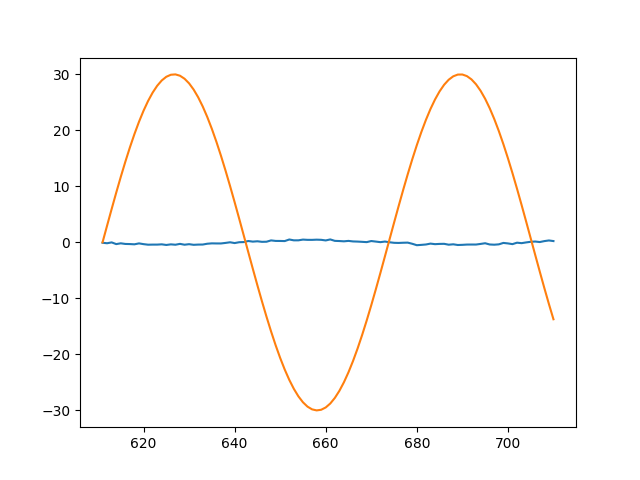# Can anybody help me with my lstm model for predicting trig function?

I am trying to predict the value of sin(x) function using nn.LSTM layer.My model:-

``````class lstm_net(nn.Module):
def __init__(self):
super(lstm_net,self).__init__()
self.lstm_layer1 = nn.LSTM(100,100)
self.layer_output = nn.Linear(100,100)
self.hidden_initialzie = (torch.zeros((1,1,100)),torch.zeros((1,1,100)))

def forward(self,x):
x , hidden = self.lstm_layer1(x,self.hidden_initialzie)
x = self.layer_output(x)
return x

``````

My data generator:-

``````x =[i for i in range(1000) ]

def random_data_generator():
y_temp = []
x_temp = []
index_start = random.choice(range(100,890))
for i in range(100):
x_temp.append(x[i+index_start])
for i in range(100):
y_temp.append((math.sin(x_temp[-1] + 0.1*i))*30)

``````

My epoch loop:-

``````for i in range(1000):
x_, y_ = random_data_generator()

output = my_model(x_)
loss = loss_function(output,y_)
loss_history.append(loss)
loss.backward()
optimizer.step()

print(loss_history[-1])
plt.plot(loss_history)
plt.show()

``````

Plotting prediction:-

``````x_ , y_ = random_data_generator()
model_prediction = my_model(x_)
y = []
for i in model_prediction:
y.append(i)
print(loss_function(model_prediction,y_))
plt.plot(x_,y)
plt.plot(x_,y_)
plt.show()
``````

I have made a function that generates random dataset for the model to train that consist of 100 sinx values and i am trying to predict next 100 sinx values but my model seems to have a very high loss.I have added my loss and prediciton graphs.

My final loss :- tensor(418.2419, grad_fn=)![predict|640x480]THIS IS MY PREDICTION GRAPH
BLUE IS MODEL PREDICTION